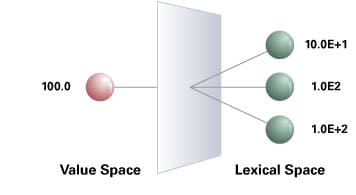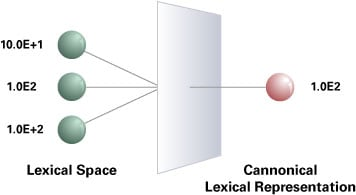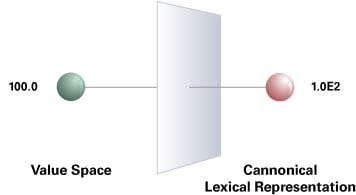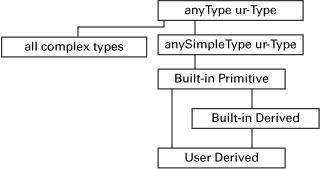Developer: XML

XML Schema: Understanding Datatypes
by Rahul Srivastava

Learn which datatypes are supported in XML Schema version 1.0, and how to use them.

Other articles in this series:
XML Schema: Understanding Namespaces
XML Schema: Understanding Structures

 Downloads for this article:Oracle XML Developer's KitOracle JDeveloper 10g (includes visual XML Schema editor)

The W3C XML Schema Datatype Specification defines numerous datatypes for validating the element content and the attribute value. These datatypes can be used to validate only the scalar content of elements, and not the non-scalar or mixed content. The text enclosed between the <opening> and </closing> element tags, and the value of the attributes are often referred to as scalar data, but it can also be a list of scalar data. These datatypes are intended for use in XML Schema definition and other XML-related documents.

Initially, Document Type Definition (DTD) was the only grammar available for validating XML instances. But DTD has only a handful of datatypes, ensuring coarse validation of the scalar data in XML via the familiar PCDATA, CDATA, and so on. XML Schema, in contrast, overcomes this limitation by providing 44 built-in datatypes. Each of these datatypes can be further customized to ensure fine validation of the scalar data. For example, the built-in datatype string can be customized to successfully validate strings and ensure they are of length 4.

• The difference between the value space, lexical space, and canonical lexical representation of the supported datatypes
• The datatypes supported in XML Schema, their classifications, and their relationships to each other
• Creation of new datatypes from the built-in datatypes using restriction, list, and union constructs
• Various constraining facets available for restricting a datatype
• How to use Oracle XDK to programmatically create and use XML Schema datatypes.

Datatype Fundamentals

Before we dive into the various types of datatypes, their usage, and the relationships between them, we need to understand datatypes as a general concept. Although XML Schema specification explains the following fundamentals about datatypes, these fundamentals are not specific to XML Schema. Rather, they are general mathematical concepts. Let's examine them in more detail.

Value Space and Lexical Space

A value space contains the maximum allowed set of values for a given datatype. Each value in the value space of a datatype is denoted by one or more literals in the lexical space of that datatype. A lexical space is the set of valid literals for a datatype.

Consider this metaphor: In the English language (and in fact in all languages), we have various words that share the same meaning. A value can be correlated to a word's meaning, and the corresponding literals can then be correlated to various different words, all having the same meaning.

For example, 100.0, 200.0, and so on are values in the value space of datatype float. The value 100.0 can be represented using multiple literals such as 10.0E+1, 1.0E2, 1.0E+2, and so on. Similarly, the value 200.0 can be represented using multiple literals such as 2.0E2, 2.0E+2, and so on. All such literals for every value in the value space of float belong to the lexical space of datatype float. (See Figure 1.)Figure 1: A value in the value space can map to many literals in the lexical space.

Canonical Lexical Representation

A canonical lexical representation is a set of literals from among the valid set of literals for a datatype such that there is a one-to-one mapping between literals in the canonical lexical representation and values in the value space. (See Figures 2 and 3.)Figure 2: Many literals in the lexical space map to exactly one literal in the canonical lexical representation.Figure 3: There is always a one-to-one mapping from the value space to the canonical lexical representation.

Canonical representations do not serve any purpose in XML Schema but are useful in other specifications that use XML Schema datatypes. For example, the XQuery/XPath datamodel uses XML Schema types as well as the canonical lexical representation to serialize a value. Therefore, when serializing a value such as 100.0, the corresponding canonical lexical representation is usedin this case, 1.0E2.

Datatypes in XMLSchema

Now that we understand the fundamental concept about datatypes in general, let's explore the datatypes available in XML Schema. Broadly speaking, the datatypes in XML Schema can be categorized as ur-Type, built-in, and user-derived (se Table 1 below) and are related to each other as shown in Figure 4.

 ur-Type anyType anySimpleType Built-in (Atomic) Primitive Derived User-Derived Restriction List Union
Table 1: XML Schema Datatype ClassificationFigure 4: Relationships between datatypes supported by XML Schema

Now, let's examine the major classificationsur-Type, built-in, and user-derivedmore closely.

ur-Type

An ur-Type is a classification that says there exists a base or root of the entire type system hierarchy in XML Schema datatypes. Any and every datatype in XML Schema has the ur-Type as its parent or ancestor. The ur-Type has a role similar to that of java.lang.Object in Java, which is the base class of all built-in and user-defined classes in that language. Similarly, the ur-type is the base of all datatypes in XML Schema. anyType and anySimpleType are the two ur-types available in XML Schema.

anyType

The anyType datatype is a concrete ur-Type, which can serve either as a complex type (non-scalar data, means elements), or as a simple type (scalar data) depending on the context. For example, here is an XML Schema using the anyType datatype:

```<?xml version="1.0" encoding="US-ASCII"?>
<schema xmlns="http://www.w3.org/2001/XMLSchema"
targetNamespace="http://mydatatypes.edu"
elementFormDefault="qualified"
attributeFormDefault="unqualified">

<element name="Currency" type="anyType" />

</schema>
```
Here is the corresponding valid instance using scalar data:
```<Currency xmlns:xsi="http://www.w3.org/2001/XMLSchema-instance"
xsi:schemaLocation="http://mydatatypes.edu ex2.xsd"
xmlns="http://mydatatypes.edu">

USD</Currency>
```
And here is the corresponding valid instance using non-scalar data:
```<Currency xmlns:xsi="http://www.w3.org/2001/XMLSchema-instance"
xsi:schemaLocation="http://mydatatypes.edu ex2.xsd"
xmlns="http://mydatatypes.edu">

<dollars>100</dollars>

</Currency>
```
anySimpleType

The anySimpleType datatype is also a concrete ur-Type, and is the parent of all built-in datatypes and ancestor of all user-derived scalar datatypes. It differs from anyType in the sense that it can hold only scalar data corresponding to any scalar datatype, whereas anyType can hold scalar as well as non-scalar data. For example, here is an XML Schema using the anySimpleType datatype:

```<?xml version="1.0" encoding="US-ASCII"?>
<schema xmlns="http://www.w3.org/2001/XMLSchema"
targetNamespace="http://mydatatypes.edu"
elementFormDefault="qualified"
attributeFormDefault="unqualified">

<element name="Currency" type="

anySimpleType" />

</schema>
```
Here is the corresponding valid instance using scalar data:
```<Currency xmlns:xsi="http://www.w3.org/2001/XMLSchema-instance"
xsi:schemaLocation="http://mydatatypes.edu ex3.xsd"
xmlns="http://mydatatypes.edu">USD</Currency>
```
And here is the corresponding invalid instance using non-scalar data:
```<Currency xmlns:xsi="http://www.w3.org/2001/XMLSchema-instance"
xsi:schemaLocation="http://mydatatypes.edu ex3.xsd"
xmlns="http://mydatatypes.edu">

<dollars>100</dollars>

</Currency>
```
In fact, if you don't specify any type for an element declaration, its type defaults to anyType, and if you don't specify any type for an attribute declaration, its type defaults to anySimpleType. In the example below, the type of element Currency defaults to anyType and the type of attribute MoreCurrency defaults to anySimpleType.
```<?xml version="1.0" encoding="US-ASCII"?>
<schema xmlns="http://www.w3.org/2001/XMLSchema"
targetNamespace="http://mydatatypes.edu"
elementFormDefault="qualified"
attributeFormDefault="unqualified">

<element name="Currency" />
<attribute name="MoreCurrency" />

</schema>
```
Built-in Datatypes

Built-in datatypes, which are defined in the W3C XML Schema Datatype Specification, must be supported by all W3C XML Schema-compliant parsers. There are two classifications of built-in datatypes: primitive and derived. The differences between the two have little relevance for the user, but we will examine them here anyway to demonstrate the mechanics and utility of datatype generation. (See the W3C's built-in datatype inheritance diagram here.)

Built-in Primitive Datatypes

Primitive datatypes are indivisible. They are not defined in terms of other datatypes; they exist independently. For example, decimal is a well-defined mathematical concept that cannot be defined in terms of any other datatypes. There are the 19 built-in primitive datatypes supported by the XML Schema Datatypes Specification:

```string
boolean
decimal
float
double
duration
dateTime
time
date
gYearMonth
gYear
gMonthDay
gDay
gMonth
hexBinary
base64Binary
anyURI
QName
NOTATION
```
For details, see Section 3.2 of the XML Schema Part 2.

Built-in Derived Datatypes

Derived datatypes, in contrast, are divisible because they are derived from the built-in primitive datatypesin other words, derived datatypes are defined in terms of other datatypes. For example, an integer is a well-defined mathematical concept that can be defined in terms of decimal with the restriction of not using the decimal point. There are 25 built-in derived datatypes supported by XML Schema Datatypes:

```normalizedString
token
language
NMTOKEN
NMTOKENS
Name
NCName
ID
IDREF
IDREFS
ENTITY
ENTITIES
integer
nonPositiveInteger
negativeInteger
long
int
short
byte
nonNegativeInteger
unsignedLong
unsignedInt
unsignedShort
unsignedByte
positiveInteger
```
For details, see Section 3.3 of Part 2 of the XML Schema spec.

User-Derived Datatypes

User-derived datatypes are the ones specified by the user in an XML Schema Definition, and are created by either restriction, list, or union. The XML Schema construct <simpleType> is used to create user-derived datatypes. Such a datatype can be named if one wants to re-use it or can be anonymous if it is to be used only once.

There has been some confusion because the specification currently categorizes list and union as user-derived datatypes. They should rather be categorized as user-defined datatypes for clarity. This confusion may be addressed in the next version of XML Schema.

User-Derived Datatype by Restriction

Every built-in datatype has a set of allowed constraining facets, which can be used to constrain or restrict that datatype, leading to the creation of a new datatype categorized as a user-derived datatype. A constraining facet is an optional property that can be applied to a datatype to constrain its "value space." Constraining the "value space" consequently constrains the "lexical space." Remember, the value space of a datatype can only be restricted and not extended. The XML Schema construct <restriction> is used to create user-derived datatypes by restricting an existing datatype with the allowed constraining facets. For example, a string of length 3 can be expressed as:

```<?xml version="1.0" encoding="US-ASCII"?>
<schema xmlns="http://www.w3.org/2001/XMLSchema"
targetNamespace="http://mydatatypes.edu"
elementFormDefault="qualified"
attributeFormDefault="unqualified">

<element name="Currency">
<simpleType>
<restriction base="string">
<length value="3" />
</restriction>
</simpleType>
</element>

</schema>
```

In the above example, an anonymous user-derived datatypethe base datatype being stringis defined along with the constraining facet, length. The same example can be written using a named user-derived datatype for re-usability:

```<?xml version="1.0" encoding="US-ASCII"?>
<schema xmlns="http://www.w3.org/2001/XMLSchema"
targetNamespace="http://mydatatypes.edu"
xmlns:tns="http://mydatatypes.edu"
elementFormDefault="qualified"
attributeFormDefault="unqualified">

<element name="Currency" type="tns:currency_type" />

<element name="MoreCurrency" type="tns:currency_type" />

<simpleType

name="currency_type">
<restriction base="string">
<length value="3" />
</restriction>
</simpleType>

</schema>
```
Following are the 12 constraining facets in XML Schema, which can be used to create a user-derived datatype from other available built-in datatypes. The constraining facets might change however depending on the base datatype:
```length
minLength
maxLength
pattern
enumeration
whiteSpace
maxInclusive
maxExclusive
minExclusive
minInclusive
totalDigits
fractionDigits
```

User-Defined List Datatype

In XML Schema a list is a sequence of homogeneous items, separated by a white space (space, tabs, carriage returns, new lines), where all the items in the list have the same datatype. It is similar to an array in Java, which is self-describing.

The XML Schema construct <list> is used to create a list datatype. For example, a list of float can be created as under:

```<?xml version="1.0" encoding="US-ASCII"?>
<schema xmlns="http://www.w3.org/2001/XMLSchema"
targetNamespace="http://mydatatypes.edu"
elementFormDefault="qualified"
attributeFormDefault="unqualified">

<element name="Currency">
<simpleType>
<list itemType="float" />
</simpleType>
</element>

</schema>
```
A list need not always be of a built-in datatype; it can also be a list of user-derived datatype. For example, a list of user-derived datatype from float, where the value is restricted from 10.0 to 20.0, can be expressed as:
```<?xml version="1.0" encoding="US-ASCII"?>
<schema xmlns="http://www.w3.org/2001/XMLSchema"
targetNamespace="http://mydatatypes.edu"
elementFormDefault="qualified"
attributeFormDefault="unqualified">

<element name="Currency">
<simpleType>
<list>
<simpleType>
<restriction base="float">
<minInclusive value="10.0" />
<maxInclusive value="20.0" />
</restriction>
</simpleType>
</list>
</simpleType>
</element>

</schema>
```
To re-use the above defined list datatype, we must name the list datatype as follows:
```<?xml version="1.0" encoding="US-ASCII"?>
<schema xmlns="http://www.w3.org/2001/XMLSchema"
targetNamespace="http://mydatatypes.edu"
xmlns:tns="http://mydatatypes.edu"
elementFormDefault="qualified"
attributeFormDefault="unqualified">

<element name="Currency" type="tns:listOfFloat" />

<simpleType name="listOfFloat">
<list>
<simpleType>
<restriction base="float">
<minInclusive value="10.0" />
<maxInclusive value="20.0" />
</restriction>
</simpleType>
</list>
</simpleType>

</schema>
```
A valid instance adhering to the above schema can hold a list of float between the range 10.0 and 20.0, both inclusive:
```<Currency xmlns:xsi="http://www.w3.org/2001/XMLSchema-instance"
xsi:schemaLocation="http://mydatatypes.edu ex5.xsd"
xmlns="http://mydatatypes.edu">10.0        12.4
15.0</Currency>
```

In the above example the items in the list are restricted to have a value from 10.0 to 20.0, but there is no restriction on the number of items in the list. If we want to restrict the number of items in the list to say 3, we can do that as follows:

```<?xml version="1.0" encoding="US-ASCII"?>
<schema xmlns="http://www.w3.org/2001/XMLSchema"
targetNamespace="http://mydatatypes.edu"
xmlns:tns="http://mydatatypes.edu"
elementFormDefault="qualified"
attributeFormDefault="unqualified">

<element name="Currency">
<simpleType>
<restriction

base="tns:listOfFloat">
<

length value="3" />
</restriction>
</simpleType>
</element>

<simpleType name="listOfFloat">
<list>
<simpleType>
<restriction base="float">
<minInclusive value="10.0" />
<maxInclusive value="20.0" />
</restriction>
</simpleType>
</list>
</simpleType>

</schema>
```
Here we used a facetlengthto restrict the number of items in the list in the above example. For datatypes derived from list datatype, regardless of the datatype of the individual itemType of list, only the following facets are allowed:
```Length
MinLength
MaxLength
Pattern
Enumeration
WhiteSpace
```
User-Derived Union Datatype

A union datatype is created by taking a union of one or more other datatypes. The XML Schema construct <union> is used to create union datatypes. For example, a union of int and float datatypes can be expressed as:

```<?xml version="1.0" encoding="US-ASCII"?>
<schema xmlns="http://www.w3.org/2001/XMLSchema"
targetNamespace="http://mydatatypes.edu"
elementFormDefault="qualified"
attributeFormDefault="unqualified">

<element name="Currency">
<simpleType>
<union memberTypes="int float" />
</simpleType>
</element>

</schema>
```
When validating the value of currency in the instance, it is first matched against datatype int. If it is not a valid int then it is matched against datatype float. If it is not a valid float either, then an error is raised. As you can see, the order in which memberTypes are declared is indeed significant, but only from a datatype validator perspective. From the user's perspective, the order of memberTypes is not significant at all.

Similar to list, a union can be of primitive datatypes as well as user-derived datatypes. For example, a union of user-derived datatypes from int and float can be expressed as follows:

```<?xml version="1.0" encoding="US-ASCII"?>
<schema xmlns="http://www.w3.org/2001/XMLSchema"
targetNamespace="http://mydatatypes.edu"
xmlns:tns="http://mydatatypes.edu"
elementFormDefault="qualified"
attributeFormDefault="unqualified">

<element name="Currency" type="tns:UnionOfIntFloat" />

<simpleType name="UnionOfIntFloat">
<union>
<simpleType>
<restriction base="int">
<minInclusive value="10" />
<maxInclusive value="20" />
</restriction>
</simpleType>

<simpleType>
<restriction base="float">
<minInclusive value="30.0" />
<maxInclusive value="40.0" />
</restriction>
</simpleType>
</union>
</simpleType>

</schema>
```
A valid instance adhering to the above schema can hold either a single int between the range 10 and 20 or a single float between the range 30.0 and 40.0, both inclusive:
```<Currency xmlns:xsi="http://www.w3.org/2001/XMLSchema-instance"
xsi:schemaLocation="http://mydatatypes.edu ex7.xsd"
xmlns="http://mydatatypes.edu">35.0</Currency>
```
When restricting a union datatype,regardless of the datatype of the individual memberTypes, only the following facets are allowed:
```Pattern
Enumeration
```
It is possible to mix and match list, union, and atomic datatypes with restrictions to define a datatype per specific requirements. For more details about constraining facets, see Section 4.1.5 of XML Schema Part 2 and Appendix B of XML Schema Part 0.

Datatype Namespaces

The datatypes that we have seen thus far are associated with the XML Schema namespace http://www.w3.org/2001/XMLSchema, which has other XML Schema constructs as well, like complexType, complexContent, group, and so on.

Because the W3C XML Schema Datatypes spec was written with the intention of not being used exclusively within XML Schema definition language, but rather also to be used by other XML-related languages, it provides a subset namespace of http://www.w3.org/2001/XMLSchema http://www.w3.org/2001/XMLSchema-datatypeswhich contains only the built-in datatypes, constraining facets, and so on needed to facilitate the use of XML Schema datatypes in other languages.

The advantage of this separation affects the XML Schema datatype validator implementation, in the sense that a standalone implementation of XML Schema datatypes is possibleas opposed to implementing the entire XML Schema Structures plus XML Schema datatypes specification.

Using Oracle XDK

Apart from validating an XML instance against the XML Schema grammar, the Oracle XML Developer's Kit (XDK) provides APIs to programmatically use the built-in datatypes, restrict them using the constraining facets, and validate a value against the schema. For example:

```import oracle.xml.parser.schema.*;
. . .

XSDSimpleType st = XSDSimpleType.getPrimitiveType(XSDSimpleType.iSTRING);
try {
//set a constraining facet on the simpleType
st.setFacet(XSDSimpleType.LENGTH, "5");
}
catch(XSDException ex1) {
System.out.println("[ERROR] Facet not supported.
"+ex1.getMessage());
}

try {
//validate value
st.validateValue("hello");
System.out.println("[SUCCESS] The value is valid.");
}
catch(XSDException ex2) {
System.out.println("[ERROR] Invalid Value. "+ex2.getMessage());
```
creates an anonymous datatype of type string and restricts it to successfully validate only strings of length 5. You can use the XDK Schema APIs to create datatypes and restrict them programmatically. See the XDK javadoc for more details.

Conclusion

Now that you understand datatypes in XML Schema and their usage, moving to other constructs of XML Schema, which define complex element content, should be much easier.

Rahul Srivastava ( rahuls@apache.org) is a senior member of Oracle Application Server development team at Oracle and is presently working in the EAI space. He has contributed in the development of the Apache open-source Xerces2-J W3C complaint validating XML Parser primarily in the area of W3C XML Schema. Rahul was also a contributor to JAXP and JSR-173 when working with Sun Microsystems as part of the Web services team.

Please rate this document:

Excellent Good Average Below Average Poor